﻿ Innovative and Time Economic Pedagogical Views in Chemical Education - A Review Article

### Innovative and Time Economic Pedagogical Views in Chemical Education - A Review Article

Arijit Das, R. Sanjeev, V. JagannadhamOPEN ACCESSPEER-REVIEWED

## Innovative and Time Economic Pedagogical Views in Chemical Education - A Review Article

Arijit Das1,, R. Sanjeev2, V. Jagannadham3

1Department of Chemistry, Ramthakur College, Agartala, Tripura, India

2Department of Pharmacy, School of Health Science, University of KwaZulu-Natal, Durban, South Africa

3Department of Chemistry, Osmania University, Hyderabad, India

### Abstract

Context based learning approaches have been presented in this article as a way to enhance student’s interest in, as well as time economic learning outcomes from chemical education. In this article 16 years research based time economic study has been presented on hydrocarbon, aromaticity and chemical bonding to cultivate the interest of teaching and learning in organic and inorganic chemistry when solving context-based chemistry problems. In this pedagogical survey, we have tried to hub twelve (12) innovative and time economic methodologies by including thirty two (32) completely new formulae in the field of Chemical Education. Concepts that usually confuse the students in the examination hall have been explained here by some innovative methods based on applied mathematics. In this context, current trends in chemistry have been highlighted by some technical methods. The review explores the results and gives implications for context-based teaching, learning and assessment.

### At a glance: Figures

1
Prev Next

• Das, Arijit, R. Sanjeev, and V. Jagannadham. "Innovative and Time Economic Pedagogical Views in Chemical Education - A Review Article." World Journal of Chemical Education 2.3 (2014): 29-38.
• Das, A. , Sanjeev, R. , & Jagannadham, V. (2014). Innovative and Time Economic Pedagogical Views in Chemical Education - A Review Article. World Journal of Chemical Education, 2(3), 29-38.
• Das, Arijit, R. Sanjeev, and V. Jagannadham. "Innovative and Time Economic Pedagogical Views in Chemical Education - A Review Article." World Journal of Chemical Education 2, no. 3 (2014): 29-38.

 Import into BibTeX Import into EndNote Import into RefMan Import into RefWorks

### 1. Introduction

In this article, we have introduced some time economic innovative methods [1-10] comprising prediction of hybridization state, bond order for diatomic homo / hetero nuclear molecules or ions having (1-20) electrons, bond-order of oxide based acid radicals, prediction of spin state using spin multiplicity value, aromatic and anti-aromatic behavior of organic compounds, evaluation of magnetic behavior of homo and hetero nuclear diatomic molecules or ions having 1-20 electrons, calculation of the number of π-bonds, σ-bonds, single and double bonds in aliphatic unsaturated open chain and cyclic olefinic hydrocarbons and alkynes etc. on conventional methods [11-23] to make chemistry time economic and more interesting. Here, we have tried to discuss them abruptly.

### 2. Innovative Thinking on the Prediction of Hybridization State

Hybridization state generally considered an operative empirical for excusing the structures of organic and inorganic compounds and their related problems.

2.1. sp, sp2, sp3 Hybridization

We know that, hybridization is nothing but the mixing of orbital’s in different ratio to form some newly synthesized orbitals called hybrid orbitals. The mixing pattern is as follows:Formula for determination of hybridization state like sp, sp2, sp3 followed the following method:

Power of the hybridization state of the central atom = (Total no of σ bonds around each central atom -1)

All single (-) bonds are σ bond, in double bond (=) there is one σ and 1π, in triple bond there is one σ and 2π. In addition to these each lone pair (i.e.no of electrons in the outermost orbit which should not take part in bond formation) and co-ordinate bond can be treated as one σ bond.

Eg.

a. In NH3, central atom N is surrounded by three N-H single bonds i.e. three sigma (σ) bonds and one lone pair (LP) i.e. one additional σ bond. So, in NH3 there is a total of four σ bonds [3 bond pairs (BPs) + 1 lone pair (LP)] around central atom N. Therefore, in this case power of the hybridization state of N = 4-1 = 3 i.e. hybridization state = sp3.

b. In H2O, central atom O is surrounded by two O-H single bonds i.e. two sigma (σ) bonds and two lone pairs i.e. two additional σ bonds. So, altogether in H2O there are four σ bonds (2 bond pairs + 2 lone pairs) around central atom O, So, in this case power of the hybridization state of O = 4-1 =3 i.e. hybridization state of O in H2O = sp3.

c. In H3BO3 :- B has 3σ bonds (3BPs but no LPs) and oxygen has 4σ bonds (2BPs & 2LPs) so, in this case power of the hybridization state of B = 3-1 = 2 i.e. B is sp2 hybridized in H3BO3. On the other hand, power of the hybridization state of O = 4-1= 3 i.e. hybridization state of O in H3BO3 is sp3.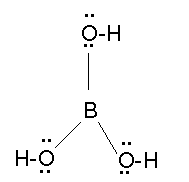d. In I-Cl, I and Cl both have 4σ bonds and 3LPs, so, in this case power of the hybridization state of both I and Cl = 4 - 1 = 3 i.e. hybridization state of I and Cl both are sp3.

e. In CH2=CH2, each carbon is attached with 2 C-H single bonds (2 σ bonds) and one C=C bond (1σ bond), so, altogether there are 3 sigma bonds. So, in this case, power of the hybridization state of both C = 3-1 = 2 i.e. hybridization state of both C’s are sp2.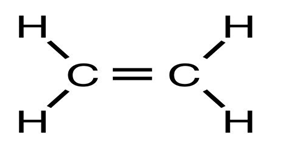2.2. sp3d, sp3d2, sp3d3 Hybridization

In case of sp3d, sp3d2 and sp3d3 hybridization state there is a common term sp3 for which 4 sigma bonds are responsible. So, in addition to 4 sigma bonds, for each additional sigma, added one d orbital gradually as follows:-

5σ bonds = 4σ bonds + 1 additional σ bond = sp3d hybridization

6σ bonds = 4σ bonds + 2 additional σ bonds = sp3d2 hybridization

7σ bonds = 4σ bonds + 3 additional σ bonds = sp3d3 hybridization

Eg:-

a. IF4-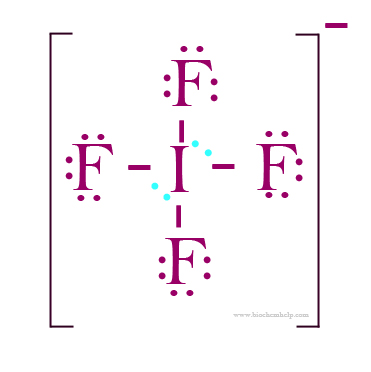: I has 7 e-s in its outermost shell, so, in this case, subtract one e- from 7 i.e. 7 – 1 = 6. So, out of 6 electrons, 4 electrons form 4 I-F bonds i.e. 4 sigma bonds and there is one LP. So, altogether there are 5 σ bonds. So, 5σ bonds = 4 σ bonds + 1 additional σ bond = sp3d hybridization

b.IF7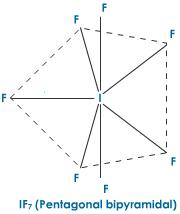7 I-F single bonds i.e. 7σ bonds = 4σ bonds + 3 additional σ bonds = sp3d3 hybridization.

c. ICl2- - I has 7 e-s in its outermost shell, so, in this case, add one e- with 7(overall charge on the compound) i.e. 07+1= 08. So, out of 08 electrons, 02 electrons form 02 I-Cl bonds i.e. 02 sigma bonds and there is 03 LPs. So, altogether there are 05σ bonds. So, 5σ bonds = 04 σ bonds + 01 additional σ bond = sp3d hybridization.

d. XeF4-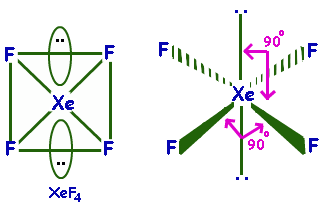Xe, an inert gas, consider 8 e-s in its outermost shell, 04 of which form 04 Xe-F sigma bonds and there is two LPs, i.e. altogether there is 06 σ bonds = 04 σ bonds + 02 additional σ bonds = sp3d2 hybridization.

In case of determination of the hybridization state by using the above method, one must have a clear idea about the outermost electrons of different family members in the periodic table as follows: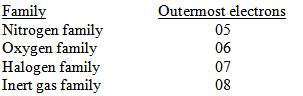And in case of cationic species you must remove electron from the outermost orbit of the central atom and incase of anionic species you must add electron with the outermost electrons of the central atom.

### 3. New Dimension for the Prediction of Bond-Order

3.1. For Diatomic Homo and Hetero Nuclear Molecules or Ions Having (1-20)e-s:
Figure 1. (B.O. vs number of electrons)

The graphical representation presented in Figure 1 shows that bond-order gradually increases to 1 in the range (0-2) electrons then it falls to zero in the range (2-4) electrons then it further rises to 1 for (4-6) electrons and once again falls to zero for (6-8) electrons then again rises to 3 in the range (8-14) electrons and then finally falls to zero for (14-20) electrons. For total no of electrons 2, 6 and 14, we use multiple formulae, because they fall in the overlapping region in which they intersect with each other.

First of all we classify the molecules or ions into four (4) types based on the total number of electrons.

i) Molecules and ions having total no of electrons within the range (1-2)

In such case Bond order = n/2; [Where n = Total no of electrons]

Eg. H2 (Total es = 2), Therefore B.O. = n/2 = 2/2 = 1

ii) Molecules and ions having total no of electrons within the range (2-6).

In such case Bond order = I 4- n I/2;

[Where n = Total no of electrons, ‘I I’ indicates Mod function i.e. the value of bond order is always positive]

Eg. Li2+(5e-s) Therefore B.O. = I 4-5 I / 2 = 1/2 = 0.5.

iii) Molecules and ions having total no of electrons within the range (6-14).

In such case Bond order = I 8-n I / 2

Eg: CO (Total es = 6+8=14), Therefore B.O.= I 8-14 I / 2 = 3

iv)Molecules and ions having total no of electrons within the range (14-20).

In such case Bond order = (20-n) / 2; [Where n = Total no of electrons]

Eg. NO (Total es = 15), Therefore B.O. = 20-15/2 = 2.5

3.2. Bond-Order Prediction for Oxide Based Acid Radicals: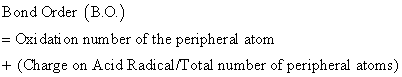Eg.

Lewis structure of ClO4-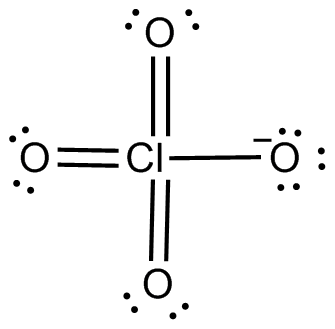ClO4- ; (Oxidation number of one Peripheral atom i.e. Oxygen = 2, Charge on acid radical = -1, Total Number of Peripheral atoms = 04), Therefore B.O. = 2 + (-1/4) = 1.75;

Lewis structure of ClO3-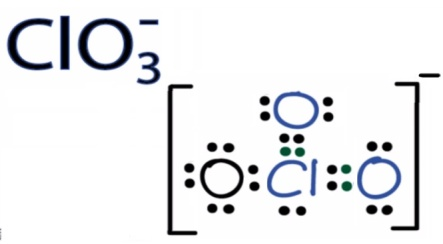ClO3-; (Oxidation number of one Peripheral atom i.e. Oxygen = 2, Charge on acid radical = -1, Total Number of Peripheral atoms = 03), Therefore B.O. = 2 + (-1/3) = 1.66;

Lewis structure of ClO2-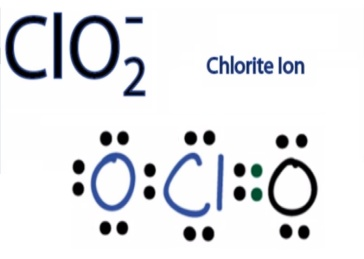ClO2-; (Oxidation number of one Peripheral atom i.e. Oxygen = 2, Charge on acid radical = -1, Total Number of Peripheral atoms = 02), Therefore B.O. = 2 + (-1/2) = 1.5;

AsO43- ; (Oxidation number of one Peripheral atom i.e. Oxygen = 2, Charge on acid radical = -3, Total Number of Peripheral atoms = 04), Therefore B.O. = 2 + (-3/4) = 1.25,

AsO33-; (Oxidation number of one Peripheral atom i.e. Oxygen = 2, Charge on acid radical = -3, Total Number of Peripheral atoms = 03), Therefore B.O. = 2 + (-3/3) = 1.0;

SO42- ; (Oxidation number of Peripheral atom i.e. Oxygen = 2, Charge on acid radical = -2, Number of Peripheral atoms = 04), Therefore B.O. = 2 + (-2/4) = 1.5;

SO32- ; (Oxidation number of Peripheral atom i.e. Oxygen = 2, Charge on acid radical = -2, Number of Peripheral atoms = 03), Therefore B.O. = 2 + (-2/3) = 1.33

PO43- ; (Oxidation number of Peripheral atom i.e. Oxygen = 2, Charge on acid radical = -3, Number of Peripheral atoms = 04), Therefore B.O. = 2 + (-3/4) = 1.25

BO33- ; (Oxidation number of Peripheral atom i.e. Oxygen = 2, Charge on acid radical = -3, Number of Peripheral atoms = 03), Therefore B.O. = 2 + (-3/3) = 1

CO32- ; (Oxidation number of Peripheral atom i.e. Oxygen = 2, Charge on acid radical = -2, Number of Peripheral atoms = 03), Therefore B.O. = 2 + (-2/3) = 1.33

SiO44-; (Oxidation number of Peripheral atom i.e. Oxygen = 2, Charge on acid radical = - 4, Number of Peripheral atoms = 04), Therefore B.O. = 2 + (- 4/4) = 1

Relation of Bond order with Bond length, Bond Strength, Bond energy, Thermal stability and Reactivity:

B.O. α 1 / Bond length or Bond distance;

B.O. α Bond strength;

B.O. α Bond Energy;

B.O. α Thermal Stability; B.O. α 1 / Reactivity

Correlation among / between Literature values of bond-distances of some oxide based acid radicals with their predicted bond order values:

Literature values of the Cl-O average bond lengths in ClO4-,ClO3- and ClO2- ; As-O bond lengths in AsO43- and AsO33- with respect to their Bond order suggest that with increasing bond-order M-O bond length (Where M = Cl, As etc.) decreases which is shown in Table 1.

### 4. Isolation of Magnetic Behavior of Homo and Hetero Nuclear Diatomic Molecules or Ions

The present study involves three new formulae by just manipulating the number of unpaired electrons (n) using mod function (based on Applied Mathematics) and by means of these n values one can easily stumble the magnetic moment values in Bohr-Magneton using spin only formula μs = √n (n+2) B.M., where B.M. = Bohr Magneton = Unit of Magnetic Moment, n = number of unpaired electrons.

First of all we classify the molecules or ions depending on the total number of electrons present in them in the following three (03) sets.

Set-1: Molecules or ions having (1-3)e-s, (3-5)e-s, (5-7)e-s, (7-10)e-s, (13-16)e-s;

Set-2: Molecules or ions having (10-13)e-s and (16-19)e-s

Set-3: Molecules or ions having 20 e-s

Then for different set we have to use three different formulae to calculate the number of unpaired electrons which have been presented in Table 2 and thus magnetic moment (μs in B.M.) can be evaluated in the following way.

#### Table 2. (Magnetic moments of homo and hetero nuclear diatomic molecules or ions)

4.1. F-1(For Set-1) - for the Determination of Number of Unpaired Electrons (n) of Molecules or Ions Having Total Number of Electrons (1-3),(3-5),(5-7),(7-10) and (13-16)e-s:

In this case, the number of unpaired electrons n = [ I (ND - total e-s) I ]

Here, ND = next digit i.e. digit next to minimum digit and ‘I I’ indicates Mod Function.

Eg:Molecules or ions having (1-3)e-s, in this case ND = 2 because here minimum digit is 1.

Eg. He2+ (3e-s), the total number of electrons will be 3, ND = 2, Hence, unpaired electron n = I (ND - total e-s) I = I (2-3) I = 1. Hence, Magnetic Moment μs = √n(n+2) B.M. = √ 1(1+2) BM = √3 BM = 1.73BM.

For the molecules or ions containing (3-5)e-s, (5-7)e-s, (7-10)e-s, and (13-16)e-s the ND value will be 4, 6, 8 and 14 respectively.

Hence, the value of n = [ I (4-total e-s) I ]; [ I (6- total e-s) I] [I (8- total e-s) I ] and [ I (14- total e-s) I ] respectively.

4.2. F-2(For Set-2) - for the Determination of Number of Unpaired Electrons (n) of Molecules or Ions Having Total Number of Electrons (10-13) and (16-19):

In this case, the number of unpaired electrons n = [ I (PD - total e-s) I ]

Here, PD = Penultimate electron digit (i.e. before last electron).

Eg: for C2- (13e-s), the total number of electrons will be 13, PD = 12

Hence, unpaired electron n = I (12 - total e-s) I = I (12-13) I = 1

Hence, Magnetic Moment μs = √n(n+2) B.M. = √ 1(1+2) BM = √3 BM = 1.73BM

For F2 (18e-s), the total number of electrons will be 18, PD = 18

Hence, unpaired electron n = I (18 - total e-s) I = I (18-18) I = 0

Hence, Magnetic Moment μs = √n(n+2) B.M. = √ 0(0+2) BM = 0 BM = Diamagnetic in nature.

4.3. F-3(For Set-3) - for the Determination of Number of Unpaired Electrons (n) of Molecules or Ions Having Total Number of Electrons 20:

In this case, the number of unpaired electrons n = [ I (20 - total e-s) I ]

Eg: for Ne2 (20e-s), the total number of electrons will be 20,

Hence, unpaired electron n = I (20 - total e-s) I = I (20-20) I = 0

Hence, Magnetic Moment μs = √n(n+2) B.M. = √ 0(0+2) BM = 0 BM = Diamagnetic in nature.

### 5. Evaluation of Spin Multiplicity Value

The formula which is generally used for the prediction of spin multiplicity value is [(2S+1), where S = Σs = total spin quantum no]. Here another innovative method has to be introduced for calculation of spin-multiplicity value and thus spin state, shown in Table 3.

#### Table 3. (Spin multiplicity value and its corresponding spin state)

First of all we should classify the species (atoms, molecules, ions or complexes) for which spin multiplicity should be evaluated into three types based on the nature of alignment of unpaired electrons present in them.

5.1. Species Having Unpaired Electrons in Upward Alignment (↑):

In this case, spin multiplicity = (n+1); where n = number of unpaired electrons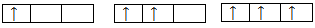Spin multiplicity = (n +1) = (1+1) = 2 (spin state = doublet); (2+1) = 3 (spin state = triplet) and (3 + 1) = 4 (spin state = quartet) respectively.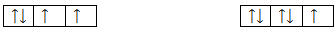Spin multiplicity = (n +1) = (2 + 1) = 3 (in this case ignore paired electrons) (spin state = triplet) and (1 + 1) = 2 (spin state = doublet)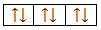Spin multiplicity = (n +1) = (0 + 1) = 1 (spin state = singlet).

5.2. Species Having Unpaired Electrons in Downward Alignment (↓):

In this case spin multiplicity = (-n+1)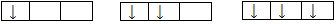Spin multiplicity = (-n +1) = (-1 + 1) = 0; (-2 + 1) = -1 and (-3 + 1) = -2 respectively.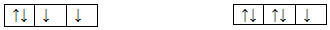Spin multiplicity = (-n + 1) = (-2 + 1) = -1( ignore paired electrons) and (-1 + 1) = 0 respectively.

5.3. Species Having Unpaired Electrons in Both Upward and Downward Alignment

In this case spin multiplicity = [(+n) + (-n) + 1]

where, n = number of unpaired electrons in each alignment. Here, (+ve) sign and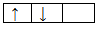Here total no of unpaired electrons = 2 in which one having upward direction (+1) and other having downward mode (-1).

Hence Spin multiplicity = [(+n) + (-n) +1] = [(+1) + (-1) + 1] = 1 (spin state = singlet)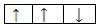Here the total no of unpaired electrons = 3 in which two unpaired electrons lie in upward (+2) and one unpaired electrons lie in downward (-1).

Hence Spin multiplicity = [(+n) + (-n) + 1] = [(+2) + (-1) + 1] = 2 (spin state = doublet)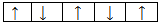Here the total no of unpaired electrons = 5 in which three unpaired electrons lie upward (+3) and two unpaired electrons lie downward (-2).

Hence Spin multiplicity = [(+n) + (-n) + 1] = [(+3) + (-2) +1] = 2 (spin state = doublet).

### 6. Identification of Aromatic and Anti-Aromatic Nature of Organic Compounds

The present study will be an innovative method involving two formulae by just manipulating the no of π bonds within the ring system and delocalized electron pair (excluding π electron pair within the ring system) with one (01).

6.1. Prediction of Aromatic Behavior:

In the first case, the compound must be cyclic, planar (i.e. all the carbon atoms having same state of hybridization) with even number of A value, where [A = πb+e-p+1(constant)], here πb = number of π bonds with in the ring system and e-p = number of electron pair outside or adjacent to the ring system i.e. if the ring contains hetero atoms (atoms containing lone pair of electrons) which can undergo delocalization and each negative charge if present may be treated as one pair of electrons.

If the value of ‘A’, for a certain organic compound comes out as even number then this compound will be treated as aromatic compound.

6.2. Prediction of Anti-aromatic Behavior

In the second case, the compound must be cyclic, planar (i.e. all the carbon atoms having same state of hybridization) with odd number of A value, where [A = πb+e-p+1(constant)], here πb = number of π bonds with in the ring system and e-p = number of electron pair outside or adjacent to the ring system i.e. if the ring contains hetero atoms which can undergo delocalization and each negative charge if present, may be treated as one pair of electrons.

If the value of ‘A’, for a certain organic compound comes out as odd number then this compound will treat as anti-aromatic compound.

6.3. In General Condition for Non-aromatic Behavior of Organic Compounds

Any compound that lacks one or more of the above features i.e. it may be acyclic / non-planar, is to be treated as non aromatic. But in this case, ‘A’ value may be even or odd number.

#### Table 4. (Aromatic, anti-aromatic and non-aromatic nature of organic compounds by calculating A value)

It is always to be noted that if the ring contains hetero atom like N, O, S etc, in this case we must count that electron pair in the evaluation of ‘A’ value which can undergo delocalization. We never count localized electron pair. Examples have been illustrated in Table 4.

There are some compounds which do not follow the above rule. Huckel’s also cannot explain the aromatic or non aromatic behavior of these compounds. These compounds have been represented in the Table 5.

#### Table 5. (Omission behavior of aromatic and non-aromatic organic compounds)

If we easily predict the nature of organic compound i.e. aromatic, anti aromatic or non aromatic then we can resolve different kind of problems regarding stability, reactivity, acidity etc. by using the following supposition.

1. Order of stability is aromatic > non aromatic > anti aromatic

2. Order of reactivity just follows the reverse order of stability as follows

Anti-aromatic > non aromatic > aromatic

3. Acidity: Stability of Conjugate base α acidity

Eg: cyclopentadienyl anion (aromatic) > cyclopentadiene (non-aromatic) > cyclopentadienyl cation (anti aromatic). Hence, cyclopentadiene (its conjugate base i.e. Cyclopentadienyl anion is aromatic in nature) is much more acidic than cycloheptatriene (its conjugate base i.e. Cycloheptatrienyl anion is anti-aromatic in nature).

### 7. Calculation of π-bonds, σ-bonds, Single and Double Bonds in Alkenes and Cycloalkenes

The molecular formula which defines a very large number of chemical structure, in this particular case, it is a herculean task to calculate the nature and number of bonds. Earlier Badertscher et al studied a novel formalism to characterize the degree of unsaturation of organic molecules. But no such work has not been taken till now to calculate the number and types of bonds in open chain olefinic system having complex molecular formulae like C176H250, C2000H2000.

Keeping this in view, a rapid method has been proposed for the calculation of number of π-bonds, σ-bonds, single and double bonds with the help of following 06 (six) completely new formulae for certain aliphatic unsaturated open chain and cyclic olefinic hydrocarbons.

7.1. For Open Chain Aliphatic Hydrocarbons:
7.1.1. Calculation of π-bonds and Double Bonds (P):

In the first case, we have to count the number of carbon atoms (X) and the number of hydrogen atoms (Y) in a given unsaturated hydrocarbon containing double bonds.

The formula to calculate the number of π bonds or double bonds for an aliphatic straight chain olefin is P= [(2X-Y)/2] + 1.

Where, X = number of carbon atoms; Y = number of hydrogen atoms and P = number of π bonds/double bonds. E.g.: In C176H250, X = 176, Y = 250, therefore P = (2 x 176 – 250)/2 +1 = 51 + 1 = 52 number of π bonds or double bonds.

7.1.2. Calculation of σ-bonds (S):

In this case, first we have to count the number of carbon atoms (X) and the number of hydrogen atoms (Y) in the given unsaturated hydrocarbon containing double bonds.

The formula to calculate the number of σ bonds for an aliphatic straight chain olefin is S = [X + Y - 1]

Where, X = number of carbon atoms; Y = number of hydrogen atoms and S = number of sigma bonds (σ-bonds).

E.g.: In C176H250, X = 176, Y = 250, therefore P = 176 + 250 -1 = 425 σ bonds.

7.1.3. Calculation of Single Bonds (A):

The total number of single bond for an aliphatic straight chain olefin is A = [(3Y/2)-2]

where A = number of single bonds and Y is number of hydrogen atoms.

E.g.: In C176H250, Y = 250, therefore A = [(3 x 250)/2] = 375 -2 = 373 single bonds. Examples have been illustrated in Table 6.

#### Table 6. (Calculation of π-bonds, σ-bonds, single and double bonds in open chain Alkene)

7.2. For Cyclic Aliphatic Olefinic Hydrocarbons:
7.2.1. Calculation of π-bonds and Double Bonds (Pc):

In the first case, we have to count the number of carbon atoms (X) and the number of hydrogen atoms (Y) in the given unsaturated cyclic olefinic hydrocarbons.

The formula to calculate the number of π bonds or double bonds for an aliphatic cyclic olefin is Pc= [(2X-Y)/2]

Where, X = number of carbon atoms; Y = number of hydrogen atoms and Pc = number of π bonds or double bonds in the cyclic olefinic system.

E.g.: In cyclooctatetraene (C8H8), X = Y = 8, therefore Pc = 16-8/2 = 4 number of π bonds or double bonds.

7.2.2. Calculation of σ-bonds (Sc):

In the first case, we have to count the number of carbon atoms (X) and the number of hydrogen atoms (Y) in the given unsaturated cyclic olefinic hydrocarbons.

The formula to calculate the number of σ bonds for an aliphatic cyclic olefin is Sc = [X + Y]

Where, X = number of carbon atoms; Y = number of hydrogen atoms and Sc = number of sigma bonds (σ-bonds) in cyclic olefinic system.

Eg: In cyclooctatetraene (C8H8), X = Y = 8, therefore Sc = 8+8 = 16 number of σ bonds.

7.2.3. Calculation of Single Bonds (Ac):

The total number of single bonds in aliphatic cyclic olefin can be calculated by using the formula Ac = [3Y/2] where Ac = number of single bonds and y is number of hydrogen atoms in aliphatic cyclic olefin.

E.g.: In cyclooctatetraene (C8H8), Y = 8, therefore Ac = 24/2 = 12 number of single bonds. Examples have been illustrated in Table 7.

### 8. Calculation of π-bonds, σ-bonds, single and Triple Bonds in Alkyne and Cycloalkyne

The number and types of bonds in open chain and cycloalkynes having complex molecular formula is a Herculean task. Keeping this in view, a rapid method has been proposed for the calculation of number of π-bonds, σ-bonds, single and triple bonds with the help of following 08 (eight) completely new formulae by just manipulating the number of carbon and hydrogen atoms by using some factors for certain aliphatic unsaturated open chain and cycloalkynes.

8.1. Open Chain Aliphatic Alkynes
8.1.1. Calculation of π-bonds (P)

In the first case, we have to count the number of carbon atoms (X) and the number of hydrogen atoms (Y) in a given unsaturated hydrocarbon containing triple bonds. The formula to calculate the number of π bonds for an aliphatic open chain alkyne, where there is one or more than one triple bonds is P= [{(2X-Y)/2} + 1]

Where, X = number of carbon atoms; Y = number of hydrogen atoms and P = number of π bonds.

E.g.: In C16H30, X = 16, Y = 30, therefore P = [{(2X-Y)/2} + 1] = [{(2 x 16 – 30)/2} +1] = 1 + 1

= 2 number of π bonds.

8.1.2. Calculation of σ-bonds (S):

In the first case, we have to count the number of carbon atoms (X) and the number of hydrogen atoms (Y) in a given unsaturated hydrocarbon containing triple bonds. The formula to calculate the number of σ bonds for an aliphatic open chain alkyne, where there is one or more than one triple bonds is S= [X+Y-1]

Where, X = number of carbon atoms; Y = number of hydrogen atoms and S = number of σ bonds.

E.g.: In C16H30, X = 16, Y = 30, therefore, S= [X+Y-1] = [16+30-1] = 45 numbers of σ bonds.

8.1.3. Calculation of Single Bonds (A)

The total number of single bond for an aliphatic open chain alkyne, where there is one or more than one triple bonds is A = [{(2X+5Y)/2} - 3]/2

Where, A = number of single bonds, X = number of carbon atoms and Y = number of hydrogen atoms.

E.g.: In C16H30, X = 16, Y = 30, therefore, A = [{(2X+5Y) / 2} - 3]/2

= [{(2x16+5x30)/2}-3]/2 = [91-3]/2 = 44 numbers of single bonds.

8.1.4. Calculation of Triple bonds (T)

In the first case, we have to count the number of carbon atoms (X) and the number of hydrogen atoms (Y) in a given unsaturated hydrocarbon containing triple bonds. The formula to calculate the number of triple bonds for an aliphatic open chain alkyne, where there is one or more than one triple bonds is T= [{(2X-Y)/2} + 1]/2

Where, X = number of carbon atoms; Y = number of hydrogen atoms and T = number of triple bonds.

E.g.: In C16H30, X = 16, Y = 30, therefore, T = [{(2X-Y)/2} + 1]/2 = [{(2 x 16 – 30)/2} +1]/2

= 2/2 = 1 triple bond.

Examples have been illustrated in Table 8.

#### Table 8. (Calculation of π-bonds, σ-bonds, single and triple bonds in open chain Alkyne)

8.2. Cycloalkynes
8.2.1. Calculation of π-bonds (Pc):

In the first case, we have to count the number of carbon atoms (X) and the number of hydrogen atoms (Y) in the given unsaturated cycloalkyne. The formula to calculate the number of π bonds for an aliphatic cycloalkyne is Pc= [(2X-Y)/2]

Where, X = number of carbon atoms; Y = number of hydrogen atoms and Pc = number of π bonds in the cycloalkyne system.

E.g.: In Cycloheptyne (C7H10), X =7, Y = 10, therefore Pc = (2x7-10)/2 = 2 number of π bonds.

8.2.2. Calculation of σ-bonds (Sc):

In the first case, we have to count the number of carbon atoms (X) and the number of hydrogen atoms (Y) in the given unsaturated cycloalkyne. The formula to calculate the number of σ bonds for an aliphatic cycloalkyne is Sc = [X + Y]

Where, X = number of carbon atoms; Y = number of hydrogen atoms and Sc = number of sigma bonds (σ-bonds) in cyclo alkyne system.

E.g.: In Cycloheptyne (C7H10), X =7, Y = 10, therefore Sc = (7+10) = 17 number of σ bonds.

8.2.3. Calculation of Single Bonds (Ac):

The total number of single bond for an aliphatic cyclo alkyne is Ac = [{(2X+5Y)/2}]/2; Where, Ac = number of single bonds in cycloalkyne, X = number of carbon atoms and Y = number of hydrogen atoms.

E.g.: In Cycloheptyne (C7H10), X = 7, Y = 10, therefore, Ac = [{(2X+5Y)/2}]/2

= [{(2x7+5x10)/2}]/2=32/2 = 16 numbers of single bonds.

8.2.4. Calculation of Triple bonds (T):

In the first case, we have to count the number of carbon atoms (X) and the number of hydrogen atoms (Y) in a given unsaturated cyclo system containing triple bond. The formula to calculate the number of triple bond is Tc= [{(2X-Y)/2}]/2.

Where, X = number of carbon atoms; Y = number of hydrogen atoms and Tc = number of triple bond.

E.g.: In Cycloheptyne (C7H10), X =7, Y = 10, therefore, Tc = [{(2X-Y)/2}]/2 = [{(2 x 7 – 10)/2}]/2 = 2/2 = 1 triple bond.

### 9. Conclusions

It may be expected that these innovative methods would go a long way to help to the students of chemistry at Undergraduate, Senior Undergraduate and Post-Graduate level who would choose the subject as their career. Experiment in vitro on 150 students at Undergraduate, Senior Undergraduate and Post-Graduate level showed that by using these new innovative methods students can save up to 30-40 mins time in the examination hall. On the basis of this, we can strongly recommend to use these new time economic interesting pedagogies.

### Acknowledgement

The corresponding author Dr. Arijit Das, wants to dedicate this review article to his student Late Uddipan Debborma (17yrs), who unfortunately breathed his last on 9th June 2014 due to heart attack.

The corresponding author Dr. Arijit Das, would be is grateful to the SERB, DST, New Delhi, Govt. of India, for their financial assistance (Sanction no – SERB / F / 5537 / 2013-14 dated 27/11/2013 and D.O. No. SB / EMEQ - 014 / 2013).

The corresponding author would be also grateful subsequently to Prof. R. N. Mukherjee, Director, IISER, Kolkata, Prof. P. K. Chattaraj, Convenor, centre for Theoretical studies, Deptt. of Chemistry, IIT Kharagpur, India,; Prof. Samar Kumar Das, School of Chemistry, University of Hyderabad, Prof. Partha Sarathi Mukherjee, Dept. of Chemistry, Indian Institute of Science, Bangalore, Prof. A. T. Khan, Head, IIT Patna, Dr.Satish Nimse, Dept. of Chemistry, Hyllym University, South Korea, Prof. G.N.Mukherjee, Dept. of Chemistry, Calcutta University, Prof. A.K.Das, Ex Vice-Chancellor of Kalyani University, Prof. Nilashis Nandi, Dept. of Chemistry, Kalyani University, W.B., India, Prof.Md.Ali, Deptt. of Chemistry, Jadavpur University, Prof. R. A. Lal, Head, Dept. of Chemistry, NEHU, Shillong, Prof.M.K.Singh and Prof.R.K.Nath, Deptt. of Chemistry, Tripura Central University for their appreciation and recognition in this regard.

Further, the corresponding author, Dr. Arijit Das, give his cordial thanks to Prof.(Dr.) Debabrata Goswami, Principal, Ramthakur College, Agartala, Tripura(w), Tripura, India, for giving him this opportunity to carry out the research work in the college. He also give his cordial thanks to other faculty members of different departments in the college for providing him valuable supporting mentality. Finally, Dr.Das would be grateful to the Honble Chief Minister of the State,Mr. Manik Sarkar and Honble Ex-Higher Education Minister, Mr. Anil Sarkar for their felicitation with ‘Certificate of Honour’ on behalf of the State ‘Tripura’, Mr.Tapan Chakraborty, Education & Higher Education Minister, Govt. of Tripura,Mr. Sekhar Datta, Chairman, tripurainfo.com and Senior Correspondent, ‘The Telegraph’ newspaper, Mr. Goutam Das, Editor, ‘Daily Desher Katha’ news Paper, Mr.Bijan Dhar, CPIM Party Secretary of Tripura, and Mr.Jayanta Debnath, MD, Tripurainfo.com for providing their valuable sustaining mentality in this regard.

### References

  Das Arijit,‘New Innovative Methods for Prediction of Hybridization State in a Very Short Time’, IJAR, 2013, 03(07), 594.In article  Das Arijit, ‘New Innovative Methods for Determination of Spin Multiplicity, Spin State and Magnetic Properties of Diatomic Hetero Nuclear Molecules or Ions in a Very Short Interval of Time’,IJAR, 2013, 03(08), 67.In article  Das Arijit, ‘New Innovative methods for prediction Bond order of mono and Diatomic molecules or ions having total number of (1-20)es in a very short time’, IJAR, 2013, 03(09), 63.In article  Das Arijit, ‘A rapid and innovative method for the easy prediction of Magnetic behavior of homo and hetero nuclear mono and diatomic molecules or ions without MOT’, IJAR, 2013, 03(10), 1.In article  Das Arijit, ‘New Methods for Prediction of Bond Order of Mono and Diatomic Homo and Hetero Nuclear Molecules or Ions Having (1-20)e-s and Oxide Based Acid Radicals Without MOT – a Rapid Innovative Approach’,IJAR, 2013, 03(11), 41.In article  Das Arijit, ‘New Methods for the prediction of Magnetic Moment of homo and hetero nuclear mono and diatomic molecules or ions without MOT - A Rapid Innovative Approach’, IJOAR, 2013, 1, 1(10), USA.In article  Das Arijit, Adhikari Suman, Paul Bijaya, Sanjeev R. and Jagannadham V., ‘A rapid and innovative method for the identification of aromatic and anti-aromatic nature of organic compounds’,WJCE, 2013, 01(01), 6-8.In article  Das Arijit, Pal Debapriya, Adhikari Suman, Paul Bijaya, Sanjeev R. and Jagannadham V.,‘Rapid calculation of the number of π-bonds, σ-bonds, single and triple bonds in aliphatic Unsaturated open chain and cycloalkynes’,WJCE, 2014, 02(01), 1-3.In article  Das Arijit, ‘Simple Thinking Makes Chemistry Metabolic And Interesting- A Review Article’, IOSR-JAC, 2014, 6(4), pp 08-15, e-ISSN: 2278-5736.In article  Das Arijit, Pal Debapriya, Paul Bijaya, Sanjeev R. and Jagannadham V., ‘Rapid calculation of the number of π-bonds, σ-bonds, single and double bonds in aliphatic unsaturated open chain and cyclic olefinic hydrocarbons’, J. Ind. Chem. Soc. 2014, (in Press).In article  Pauling.L., ‘J.Am.Chem.Soc.’, 1931, 53(4), 1367-1400.In article  “Spectroscopy. Molecular Orbitals and Chemical Bonding”, Nobel Lectures, Chemistry 1963-1970, Elsevier Publishing Company, 1972-1966.In article  Hall, George G. Lennard-Jones Paper of “Foundations of Molecular Orbital Theory”, Advances in Quantum Chemistry, 1929, 22. Bibcode: 1991AdQC…22…1H.In article  Lee. J.D., ‘Concise Inorg. Chem,’; 5th ed., Wiley: India and Oxford, 2009, 944 and 109-112.In article  Douglas. B., Mcdaniel. D. and Alexander. J., ‘Concepts and Models of Inorg. Chem.’; 3rd ed. Wiley: India, 2007, 157 and 38.In article  Cotton. F.A., Wilkinson. G. and Gaus. P.L.(2007), ‘Basic Inorg. Chem.’, 3rd ed., Wiley: India, 2007, 107, 523 & 111.In article  Mahan. B.M. and Meyers.R.J., ‘International Student Edition University Chemistry’, 4th ed., 1998, 599-603.In article  Finar I.L, Organic Chemistry, Vol-1, Pearson, 6th ed., 2004, 1, 532, 577-580.In article  Morrison R.T. and Boyd R.N., 7th ed., Organic Chemistry, Pearson, 7th ed. 208.In article  Solomons T.W.Graham and Fryhle C.B., Organic Chemistry, Wiley: India 10th ed., 2009, 2012, 150, 632-639.In article  Smith J.G.,Organic Chemistry, 2nd ed., 2008, 616-619.In article  March Jerry, Advanced Organic Chemistry: Reaction, Mechanisms and Structure, 3rd ed., Willy: New York, 1985.In article  Martin Badertscher, Kaspar Bischofberger, Morton E. Munk, and Erno Pretsch, ‘A Novel Formalism To Characterize the Degree of Unsaturation of Organic Molecules’, J.Chem.Inf. Comput. Sci. 2001, 41, 889-893.In article CrossRef